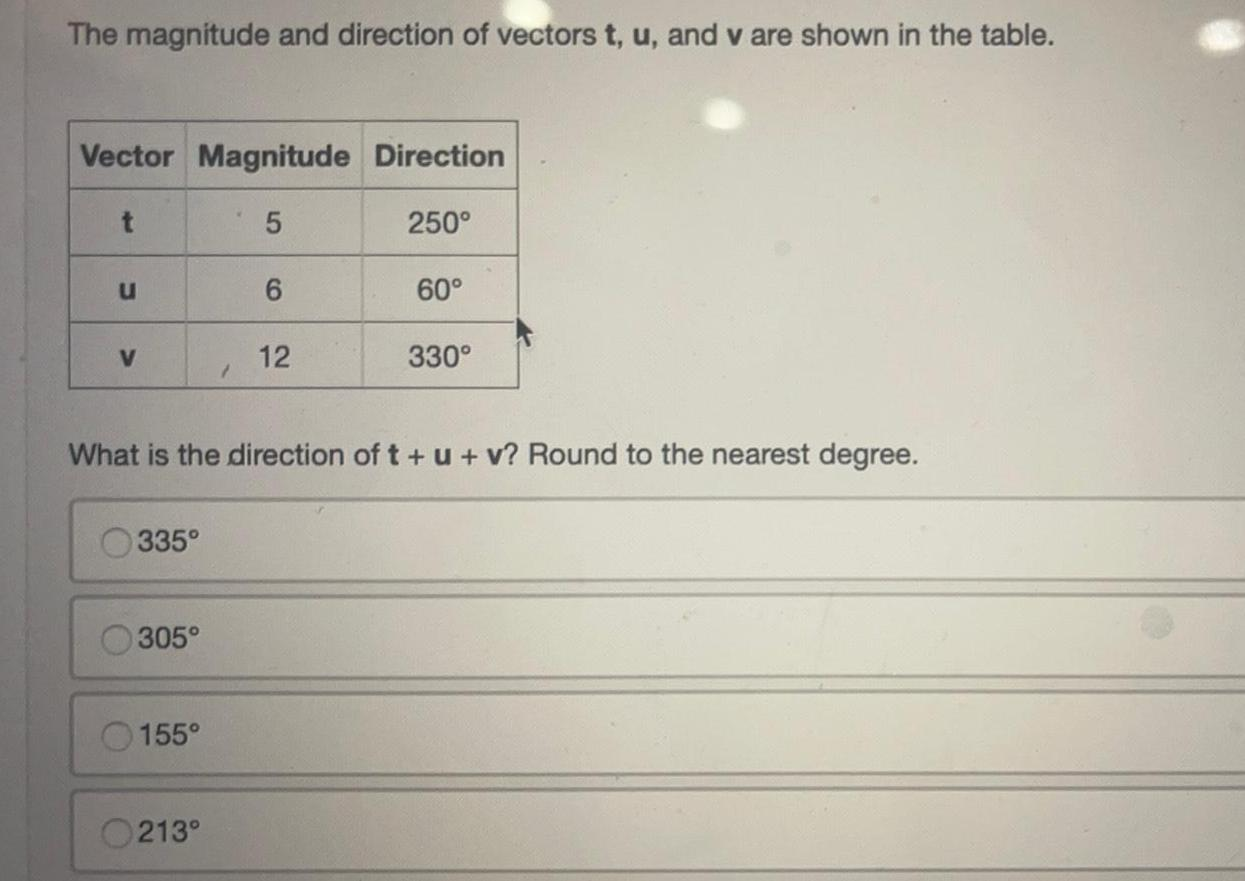Math
Vectors
The magnitude and direction of vectors t u and v are shown in the table Vector Magnitude Direction 5 250 t u V 335 305 155 1 0213 6 What is the direction of t u v Round to the nearest degree 12 60 330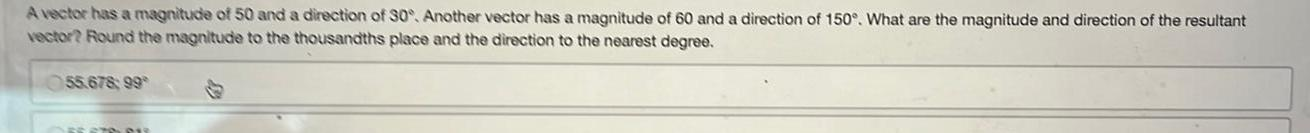Math
Vectors
A vector has a magnitude of 50 and a direction of 30 Another vector has a magnitude of 60 and a direction of 150 What are the magnitude and direction of the resultant vector Round the magnitude to the thousandths place and the direction to the nearest degree 55 678 99 FESTO PU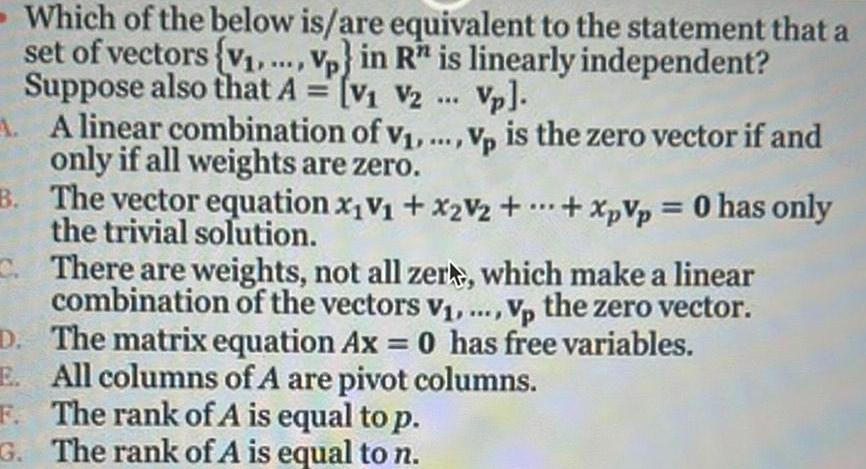Math
Vectors
Which of the below is are equivalent to the statement that a set of vectors v Vp in R is linearly independent Suppose also that A V V2 Vp A A linear combination of v Vp is the zero vector if and only if all weights are zero B The vector equation x V x2V2 XpVp 0 has only the trivial solution C There are weights not all zer which make a linear combination of the vectors V Vp the zero vector D The matrix equation Ax 0 has free variables E All columns of A are pivot columns The rank of A is equal to p F G The rank of A is equal to n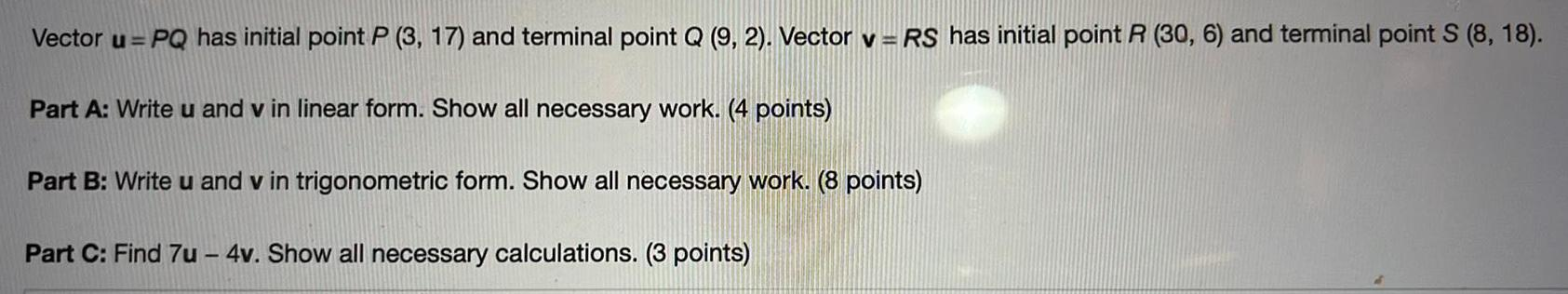Math
Vectors
Vector u PQ has initial point P 3 17 and terminal point Q 9 2 Vector v RS has initial point R 30 6 and terminal point S 8 18 Part A Write u and v in linear form Show all necessary work 4 points Part B Write u and v in trigonometric form Show all necessary work 8 points Part C Find 7u 4v Show all necessary calculations 3 pointsMath
Vectors
Vectors u v and w are described in the table Vector Magnitude Direction 35 40 13 3 W 40 What are the magnitude and direction of u v w Round the magnitude to the thousandths place and the direction to the nearest degree 38 675 12 38 675 348 40 353 12 180 310 40 353 348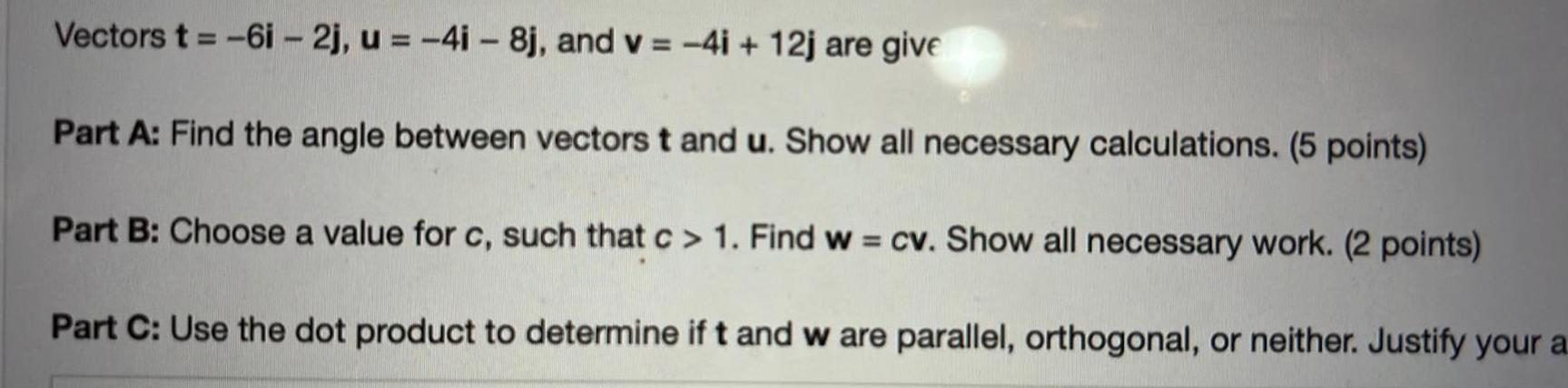Math
Vectors
Vectors t 6i 2j u 4i 8j and v 4i 12j are give Part A Find the angle between vectors t and u Show all necessary calculations 5 points Part B Choose a value for c such that c 1 Find w cv Show all necessary work 2 points Part C Use the dot product to determine if t and w are parallel orthogonal or neither Justify your a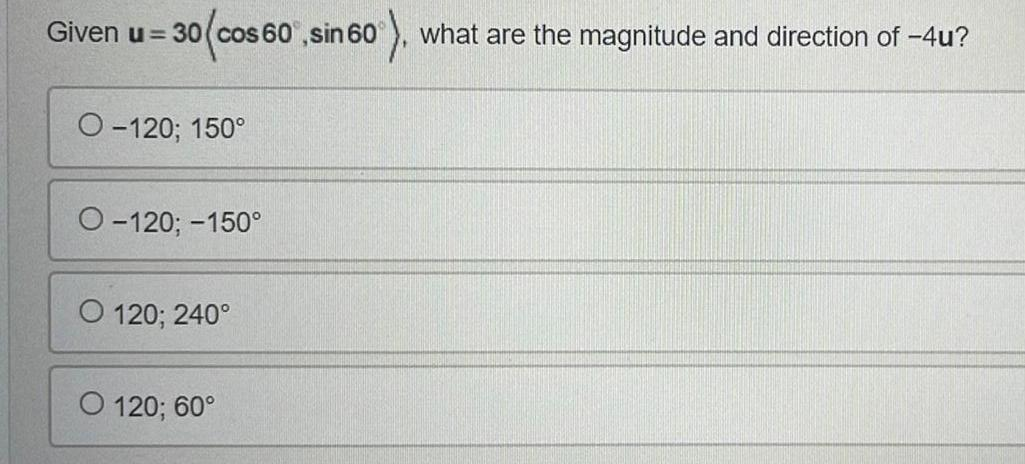Calculus
Vectors
Given u 30 cos 60 sin 60 what are the magnitude and direction of 4u O 120 150 O 120 150 O 120 240 O 120 60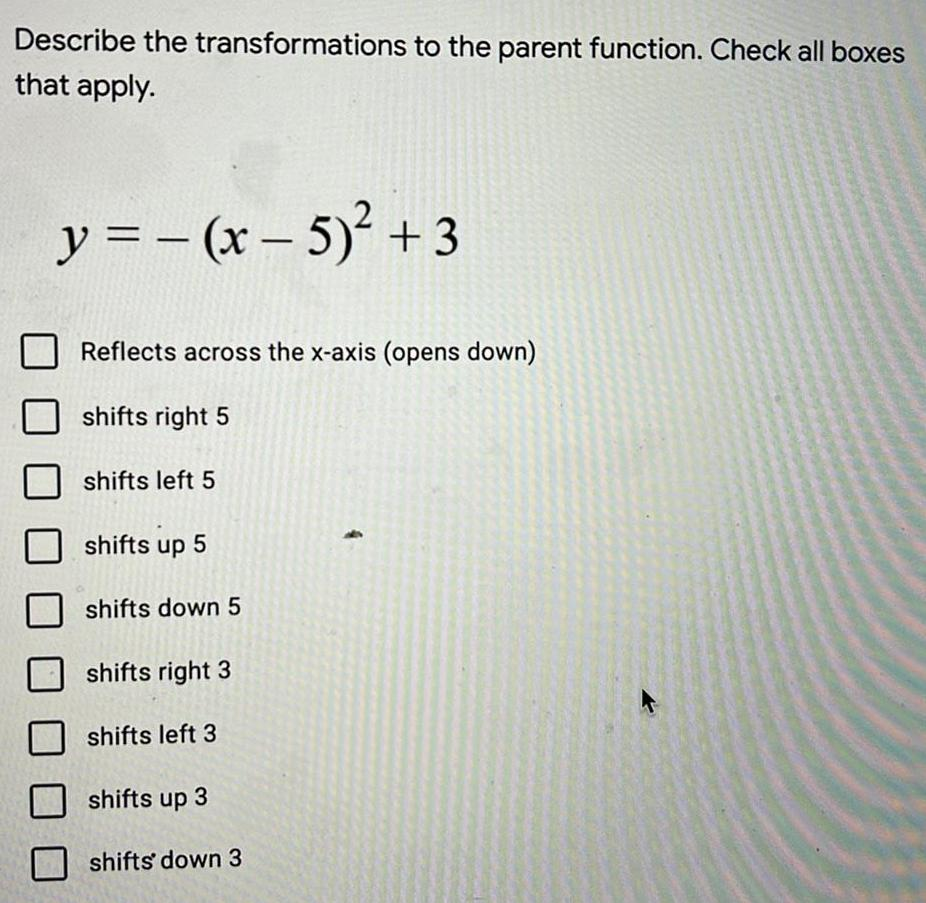Math
Vectors
Describe the transformations to the parent function. Check all boxes that apply. y = -(x - 5)² +3 Reflects across the x-axis (opens down) shifts right 5 shifts left 5 shifts up 5 shifts down 5 shifts right 3 shifts left 3 shifts up 3 shifts down 3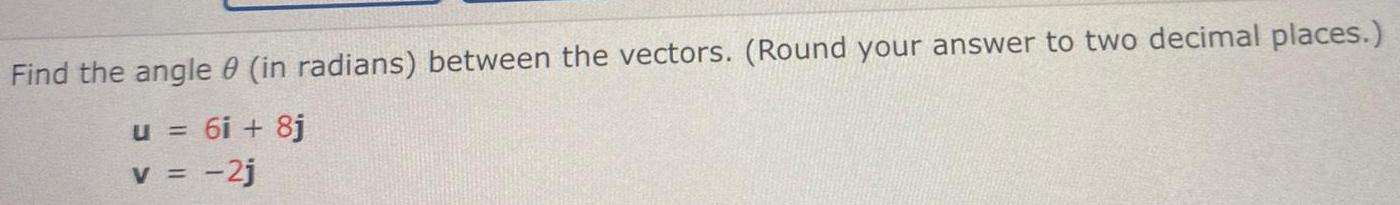Math
Vectors
Find the angle 0 (in radians) between the vectors. (Round your answer to two decimal places.) u = 6i + 8j v = -2j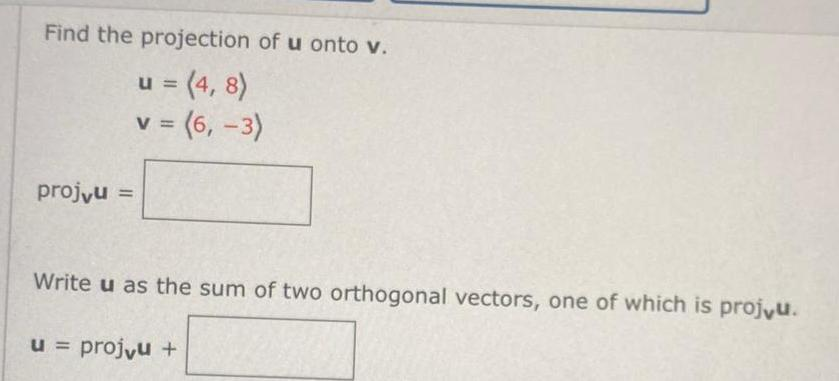Math
Vectors
Find the projection of u onto v. u = (4,8) v = (6,-3) Write u as the sum of two orthogonal vectors, one of which is projvu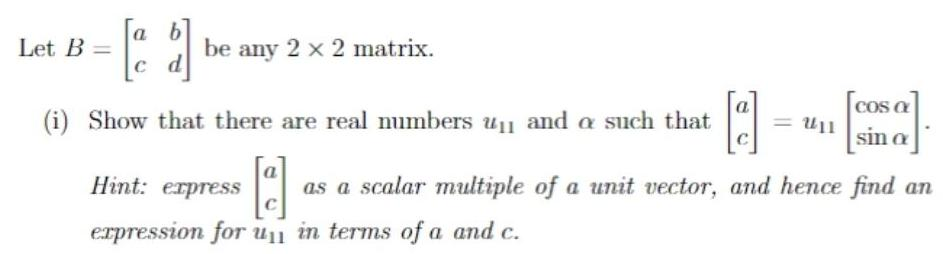Math
Vectors
Let B = be any 2 x 2 matrix. and a such that (i) Show that there are real numbers as a scalar multiple of a unit vector, and hence find an expression for u in terms of a and c.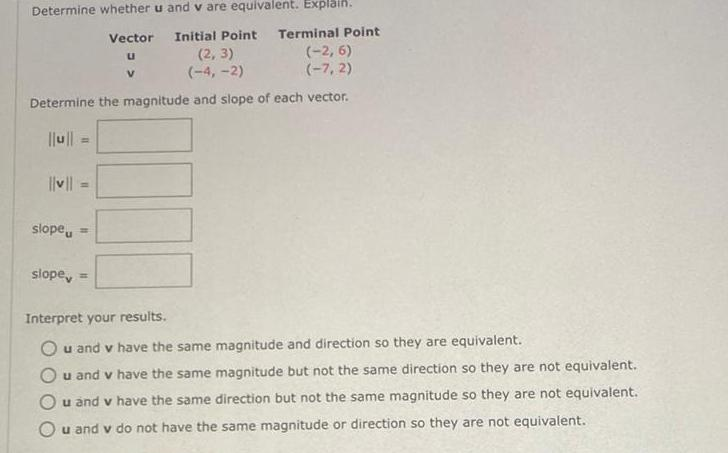Math
Vectors
Determine whether u and v are equivalent. Explain. Vector Initial Point Terminal Point Interpret your results. u and v have the same magnitude and direction so they are equivalent. u and v have the same magnitude but not the same direction so they are not equivalent. u and v have the same direction but not the same magnitude so they are not equivalent. u and v do not have the same magnitude or direction so they are not equivalent.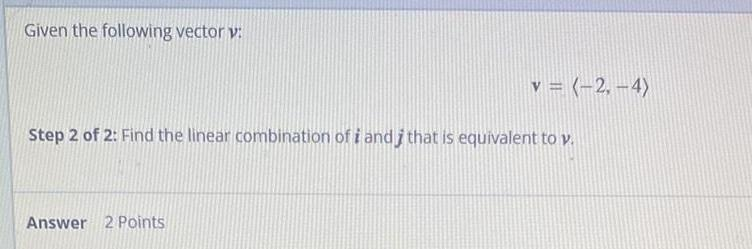Math
Vectors
Given the following vector v: v = (-2,-4) Step 2 of 2: Find the linear combination of i and i that is equivalent to v.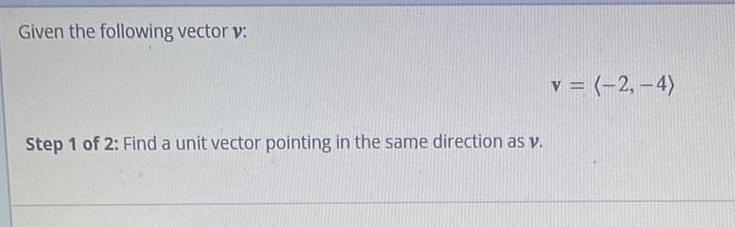Math
Vectors
Given the following vector v: Step 1 of 2: Find a unit vector pointing in the same direction as v. v = (-2,-4)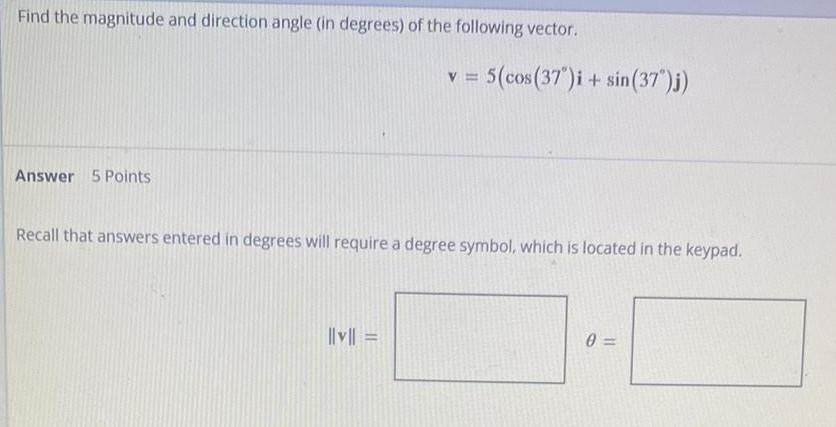Math
Vectors
Find the magnitude and direction angle (in degrees) of the following vector. Answer 5 Points v = 5(cos(37°)i + sin(37")j) Recall that answers entered in degrees will require a degree symbol, which is located in the keypad. |||v|| = 0=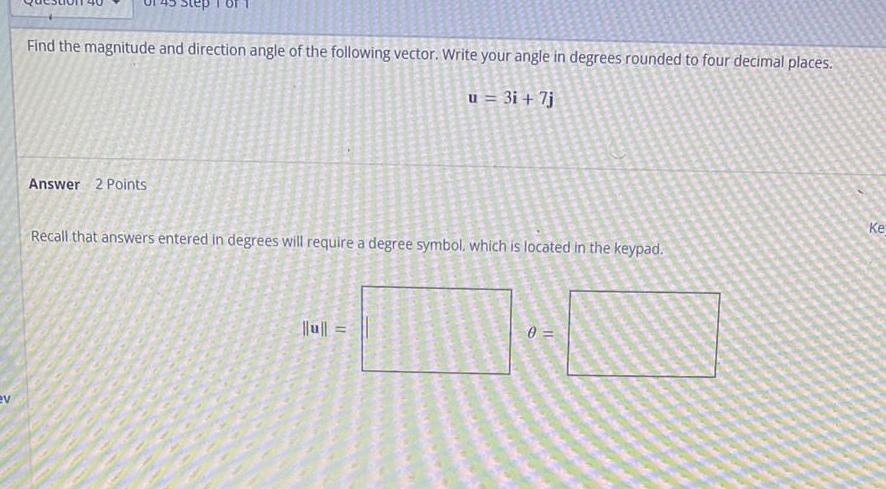Math
Vectors
Find the magnitude and direction angle of the following vector. Write your angle in degrees rounded to four decimal places. u = 3i + 7j